# The logic, definitions and math used to develop this calculator...

The following explains the logic I used to develop QuickGoldTally. I am a student of engineering, and though I have completed several courses in math and physics, I am not immune to making mistakes. For this reason, I’ve also provided my math in this document for your inspection.

Using some simple gold panning techniques, George "Buzzard" Massie, a well-known miner, once found traces of gold in fifty-pound bags of sand purchased at Home Depot. Jeff Williams, another gold miner best known for his "Ask Jeff Williams" online gold mining productions, shows us, during his video “Gold in Home Depot Sand!” that he too finds gold the same way. I did some fact-checking online and found nothing that disputes the idea of finding trace amounts of gold within sand. Finding gold in something as commonplace as sand naturally piqued my interest. However, in the video, no one ever mentions how much the gold found is worth. I wondered if a person could somehow price the gold just by looking at it.

I checked online for something that might help a person visually ballpark an estimate for the market price of gold found while panning, but found no information on this topic. So I figured I could produce some sort of visual approximation method. In order to facilitate this, I developed the calculator found on this website.

The calculator determines market price just as any scrap gold calculator would after the karat and mass are provided. But, the calculator can also determine an approximate volume (a size) for the gold when the appropriate alloy type is selected (whether it is copper or silver). The calculator can reverse-calculate a mass instead by entering an estimated size, karat, and alloy type for the gold. The calculator can also determine market price from a karat calculated after an alloy type, size and mass are entered.

If you want to skip directly to the calculator for estimating a value by eye, you’ll need to have decided what type of impurity is involved (the alloyed material within the gold). You’ll also need to have chosen a karat, as well an estimated size for the particles in question, based on the shape of a cube. The karat and alloy type can come from prior finds from the location where you found the gold, (using previous finds as a best guess, basically). With regard to the calculator, the estimated size of the particle is broken down into several convenient size-categories available from a drop down selector. Or you can manually enter a value yourself. The size (volume) is in cubic millimeters.

The top of the entry screen allows you to enter the current price of gold in dollars per gram. Or, if the connection to the gold API (Application Program Interface) is up and running, this field may have been populated automatically.

My logic would be familiar to people who have studied the works of Archimedes. Archimedes was the first person able to find gold-content in a crown that was a blend of both silver and gold. Archimedes realized that silver has a lower density than gold. He also knew that for two equivalent-weight metals, having different densities, the less dense metal takes up more space. (If each metal uses the same amount of space, the less dense metal would be lighter.)

The discovery that Archimedes specifically made himself was how to determine volume for oddly-shaped objects like crowns. For more information about Archimedes’ discovery, please read, “Eureka! The Story of Archimedes and the Golden Crown” available online.

The terms that should be understood for this topic are volume, mesh, acceleration, mass, weight, density, percent, karat, and value. Knowing the differences between percent by volume and percent by mass, and knowing how to appropriately convert units will be helpful as well. Detailed definitions for these terms are available by clicking the underlined words above. Understanding algebra to an intermediate level will be necessary for understanding the rest of this document.

Some of the pricing tools available online describe gold’s value based on a density defined as grams per cubic centimeter, which are many orders of magnitude larger than the scale we’ll be discussing for individual dust particles.

Let’s start by converting cubic centimeters to cubic millimeters:

One cubic millimeter is 1/1000th the volume of one cubic centimeter. This is known by seeing that one cubic centimeter is a cube made with 10 millimeter sides: 10 mm x 10 mm x 10 mm equals 1,000 cubic millimeters arranged inside a one centimeter cube.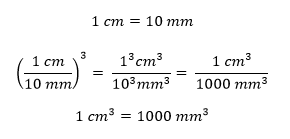Pure gold is stated as having a known density of 19.282 grams per cubic centimeter. Since one cubic millimeter is 1/1000th the volume of one cubic centimeter, one cubic millimeter of pure gold contains 0.019282 grams gold, via moving the decimal over three spaces as a means for dividing 19.282 by one thousand: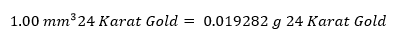September 23, 2016, pure gold had a value of \$43.10, or 4,310 cents per gram.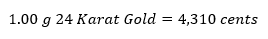In order to determine a particle’s value, we are going to ultimately need the particle’s total mass. But since we are working with visual estimates, this may not seem possible. Don’t worry, though. The particle’s total mass can be revealed mathematically using karat, volume and alloy-type. A value can then be calculated by multiplying the total calculated mass against the karat and the number of cents per gram that gold is currently worth.

For example, once we’ve determined the total mass of one cubic millimeter of 23 karat gold, (alloyed with copper), we’d take its mass and multiply it against the 23-karat ratio and then multiply that against pure gold’s monetary value of 4,310 cents per gram, equaling about seventy-six cents: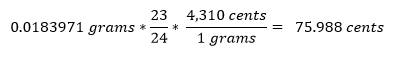The karat and alloy are presumed based upon prior mining discoveries in the area where the gold was found, while volume is visually estimated. For the sake of convenience we’ll use the term "impurity" to refer to the alloyed materials, copper and/or silver.

Now, let’s look at how we can find the particle’s total mass from karat, volume and alloy type:

We likely don’t know the exact karat right off when we first find it out in the field. However, we may know from experience what the alloy and karat typically are for the region where the gold came from. We can further assume that the golden particle will be less dense and will have a bigger volume than if it had been made entirely from pure gold to start with.

We’ll need some sort of an accurate estimate for the total volume of the particle, and we’ll create a mathematical variable for the unknown total mass at this point. The mass term we are building here will be used later to determine value, as previously stated above.

We start with a reasonable number for the total volume and a variable for the unknown mass: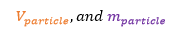The particle’s gold-content is likely near enough to one of the many frequently-seen mixtures of gold (stated in karats) used to determine a nugget’s value. The definition of karat: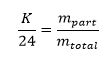Since karat is based upon mass, you’d think after getting the formula that defines karat we’d want another formula that is specifically solved for mass in order to immediately substitute between them. Instead, we are first going to solve the density formula into a formula for volume. This may sound about as sensible as hiring a dietician who wants to discuss your height rather than your weight, but it actually makes sense since all we really have at this point is an estimated volume, based upon our visual estimate of the particle’s size.

We know that the total volume of our estimate of the particle’s size has to match the sum of both the volume of the impurity and the volume of the gold blended within: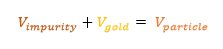Also, we know we can find the density of the impurity (either copper or silver) and the density of gold by looking these up. The formula for density is written in terms of mass divided by volume.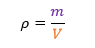Since we know that the particle’s total volume is merely the combined volume of both the impurity and the gold, we can solve the standard density formula into a formula that describes volume, written in terms of mass and density. This way, we will substitute the volume for the type of impurity and the volume of the amount of gold for statements written in terms of mass and density instead.

From there, we will determine how much mass is dedicated to pure gold and how much mass is dedicated to the impurity using karat along with the densities involved. We will then sum of our mathematically-determined individual masses to get a value for the particle’s total mass. This may seem a little odd since we don’t know the mass nor the volume of either the impurity or the gold. But, by using algebra, we can build formulae for the individual unknown portions once we have enough equations.

We are looking for two unknown portions based upon the known densities of gold and the type of impurity (either silver or copper). Each portion having two unknown qualities: a pure gold portion with its mass and volume and another portion, if any, with its mass and volume too. So to start, we’ll need four equations:

The first equation discusses karat. One twenty fourth karat equals the gold content in the total particle as a ratio: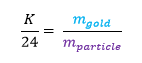The total mass of the particle is, of course, the mass of the impurity combined with the mass of the amount of gold involved. So, we can rewrite the mass of the entire particle as the sum of the masses for the impurity and the gold: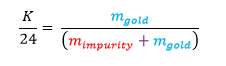Solve specifically for karat: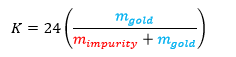The second equation is density, which is mass divided by volume: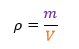Because we want to end up in terms of mass, because we want to follow our percent by mass motivation, and also in terms of density, since we can easily look those up, we need to rewrite the above density equation into an equation for volume instead.

Multiply the above equation by V on both sides, and then divide out ρ on both sides, yields a general formula for volume in terms of mass divided by density, rather than mass divided by volume: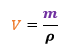The third equation is the volume of the impurity in terms of mass and density: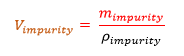And the fourth equation is gold volume in terms of mass and density: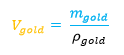Naturally, the volume of the impurity added to the volume of gold equals the total volume of the entire particle. And the total volume of the particle is something we’ve closely estimated.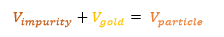We can now substitute volume for the mass/density arrangements.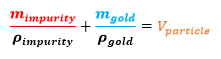Restating it slightly: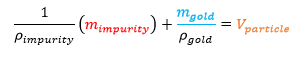Now working with the equation for karat, solving for both the mass of the impurity and gold in order to facilitate further substitution: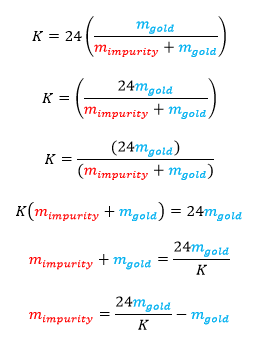We factor out mass of gold such that we are left with a formula for the mass of the impurity in terms of karat and the mass of gold: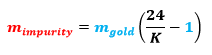Remember, we started with the following equation, substituting into it mass and density: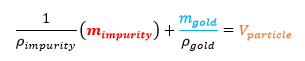Now, putting the new formula for the mass of the impurity into the summation of volume that has been rewritten in terms of mass and density, below: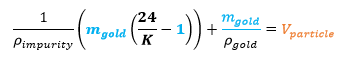Factor out the common mass of gold after surrounding the entire term with parenthesis: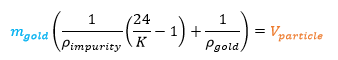Divide out the entire term contained within parenthesis such that we are left with a formula that is solved for the mass of gold: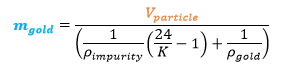Previously, we created a formula for the mass of the impurity in terms of the mass of gold:The total mass of the particle is merely the sum of the mass of the gold and the impurity: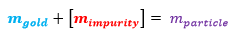Substitute in each mass formulae where appropriate, into the equation immediately above: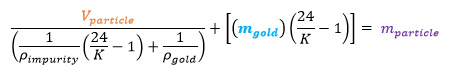Further substitute in what the mass of gold is again after including more parenthesis: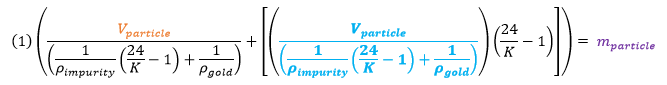Factor the now common mass of gold terms into where the number one was, above: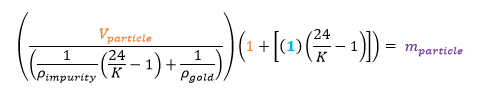Clean up resulting terms of one: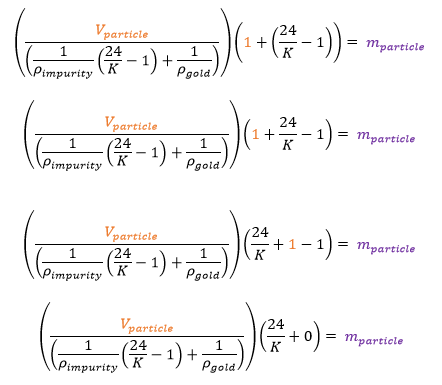And we now have a concise formula for estimating the mass of a particle once karat, volume, and densities for the particle are estimated and inserted: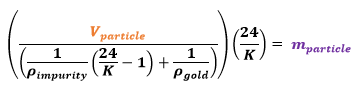Working with the above formula and the other known quantities, it is now possible to get a number for the mass merely from an estimated karat, volume and the densities involved.

Or, given that you might have somehow managed to measure both the particle’s volume and weight in grams beforehand and are actually looking for its real karat value instead, we should be able to discern a formula for what karat value is instead of deciding the karat first. We’ll look at that in a moment. For now, continuing with the mass of the particle for a given karat value, we will determine the monetary value of our particle. If we obtain the price in cents per gram for 24 karat gold, we can multiply the mass of the particle in grams against this price per gram to achieve the total value of the particle in cents.

Previously we stated the densities as grams per cubic centimeter. Given that a cubic millimeter is one thousand times smaller than a cubic centimeter, we’d divide the density down a thousand times to work in quantities around cubic millimeters. So, pure gold goes from 19.282 grams per cubic centimeter to 0.019282 grams per cubic millimeter; moving the decimal place over three times. And, if using copper as the impurity, the density of copper goes from being 8.950 grams per centimeter to 0.00895 grams per millimeter.

For example, if we think we are working with a particle possibly being 21 karats, alloyed with copper, and have visually estimated the volume of the particle as being 0.015625 cubic millimeters, (the thickness of a fingernail) we have: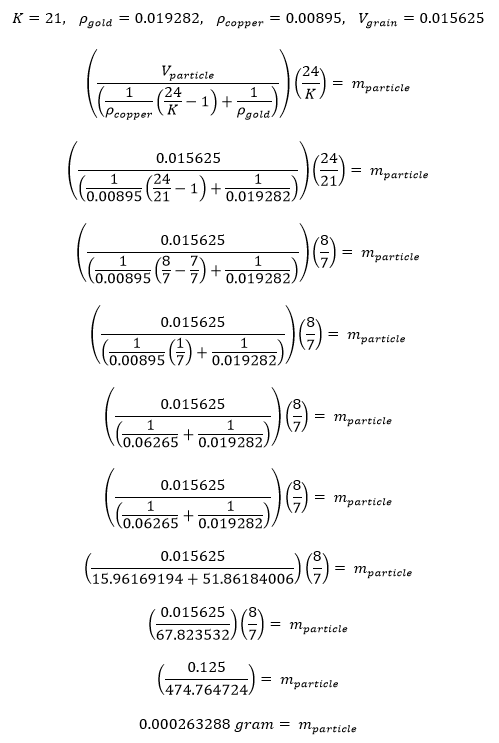Meaning we’d expect the mass for a 21 karat particle that is alloyed with copper to be around .000263288 grams if the volume for the particle was .015625 cubic millimeters.

If we further knew that for every gram of pure gold we’d earn 4,310 cents, we could then determine what our particle, being 21 karats and 0.015625 cubic millimeters, is worth in terms of cents.

First, we’d need to get the amount of pure gold we have in the particle. This is figured by using the karat formula, dividing 21 by 24, which gives us 0.875 (87.5 % percent) pure gold. Applying this to the calculated total mass tells us the amount of pure gold that there is in the particle. Multiplying the amount of pure gold against the going price gives us a value.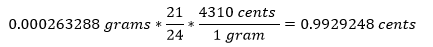So if each side of a tiny cube of golden alloy measured one quarter millimeter, we’d find its volume as ¼ mm x ¼ mm x ¼ mm which is 0.015625 cubic millimeters. And due to its density (mass/volume), the particle was determined to be 87.5 % gold and 12.5 % copper (21 karat). Therefore, its value would be right around one penny as a crude estimate.

Otherwise, if the same particle had been pure gold, it would have continued to have had the same volume of 0.015625 cubic millimeters, but its mass would have been closer to 0.000301281 grams instead of 0.000263288 grams, (it would be heavier) giving us a different density and a clue to its purity. So in this way, by noticing the specific volume and mass for each particle, it is possible to closely determine the karat value of a given particle (the percent of pure gold there is in each particle)

Now let’s develop a formula for karat based upon the mass and volume of the particle.

Starting with our formula for finding the particle’s mass, we’ll solve for K: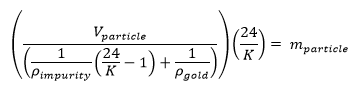Distributing the smaller fraction into the larger, yields: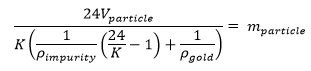Divide and multiply each side such that we flop the mass and karat terms: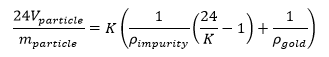Distribute K: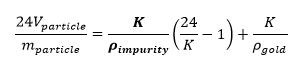Distribute the entire K term into parenthesis: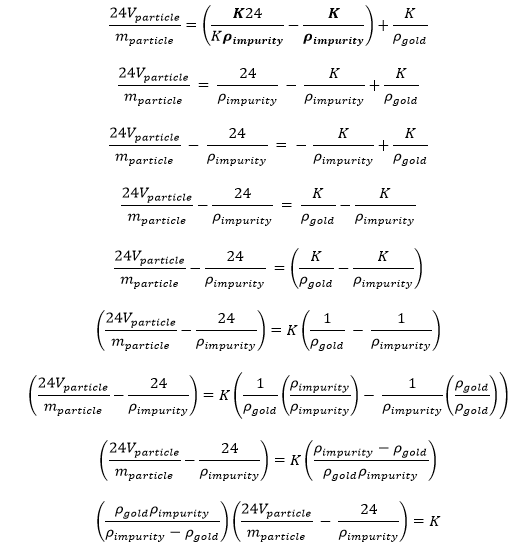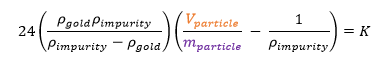Meaning if we know the total mass of the particle, in grams, and the total volume of the particle, in cubic millimeters, we can reveal the karat value based upon the densities of the materials involved, just so long as the density of the impurity is closely matched.

In other words, if it is assumed that the karat for the region where the gold was found is typically 19 karat and the alloyed material is typically silver, we could use the measured mass and volume of the particle to verify this karat value. In the formula above, one would merely need to use the density of silver for the density of the impurity.

So-called "dust" can be unthinkably small. Some particles of dust can be 30 trillion times smaller than typical pieces of sand making it very hard to quantify value for such a particles of gold.

To estimate the return on a volume of sand, we'll use Jeff William's video about the amount of gold discovered in bags of sand purchased at Home Depot. It is not known if there would be a difference between using natural sand found along a riverbank verses using sand from Home Depot, but, for now, we'll assume that there is no difference.

In Jeff's video, he works three 50-pound bags and seems to find two sand-sized pieces of gold (35 mesh) along with what looks like maybe 30 to 50 tiny particles having maybe the thickness of a piece of thread or maybe as much as the thickness of a finger-nail. We’ll say there are 40 such pieces.

Developing a mid-range estimate by using the average gold value of 18 karats and guessing that the smaller particles were somewhere between thread-sized and finger-nail sized, (between 120 and 60 mesh) it looks as though Jeff banked about 28 cents worth of gold, or maybe slightly more: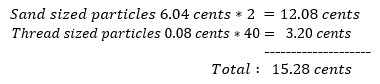And if we include an estimate where the thread-sized particles are closer to fingernail-sized: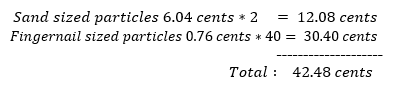Average these two estimates to get our estimate near 28 cents: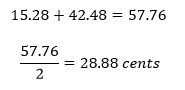If we believe that an acceptable daily wage of around \$100 per day is reasonable take-home pay, we can estimate the volume of sand that one must process in order to make this sort of wage.

We are using the idea that 150 pounds of sand yield 28 cents worth of gold (free sand obtained out in nature somewhere, and not obtained by purchasing sand from Home Depot):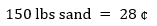And we'll convert \$100 of take-home pay into cents as 10,000 cents for a day’s work.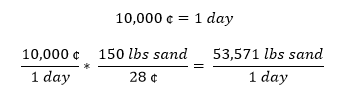Meaning a person would need to process 53,571 pounds of sand per day to make one hundred dollars. Printed on each bag of sand from Home Depot are the words, “0.33 cubic feet”. To convert pounds into cubic feet, we can use this printed information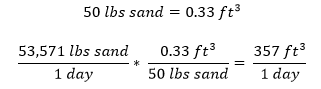Equaling 357 cubic feet of sand per day to be processed.

There are 27 cubic feet within a cubic yard. So, divide the total cubic feet by this cubic-yard factor to obtain the total cubic yards of sand to be processed.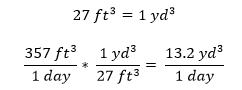Therefore, 13 to 14 cubic yards of sand must be properly processed by one person each day for a low to standard living wage for that person of just \$100 per day. This is horribly difficult, maybe even impossible. An industrial dump truck can carry 15 cubic yards. One person cannot likely process most of a dump truck’s worth of sand all by themselves in one day without a lot of expensive, giant equipment. Therefore, this becomes a project for a company that can process large amounts of sand. The draw back being the capital investment if the sand yields nothing. It would be quite a gamble. Still, the novelty of finding small amounts of gold in sand may be worth the slight entertainment value for an individual who is merely experimenting a little. Just be careful not to expect too much!

QuickGoldTally | instructions | disclaimer | technical dissertation | volume | mesh | acceleration | mass | weight | density | percent | karat | value | comments